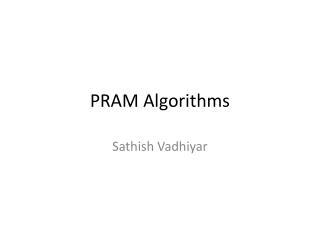DownloadDownload PresentationPRAM Algorithms

# PRAM Algorithms

Download Presentation## PRAM Algorithms

- - - - - - - - - - - - - - - - - - - - - - - - - - - E N D - - - - - - - - - - - - - - - - - - - - - - - - - - -
##### Presentation Transcript

2. PRAM Model - Introduction • Parallel Random Access Machine • Allows parallel-algorithm designers to treat processing power as unlimited • Ignores complexity of inter-process communication • Consists of control unit, global memory, and an unbounded set of processors, each with own private memory • An active processor reads from global memory, performs computation, writes to global memory • Execute in SIMD model • PRAM algorithm can be a suitable basis for the design of a parallel program targeted to a real machine

3. Different Models • Various PRAM models differ in how they handle read or write conflicts • EREW – Exclusive Read Exclusive Write • CREW – Concurrent Read Exclusive Write • CRCW • COMMON – All processors writing to same global memory must write the same value • ARBITRARY – one of the competing processor’s value is arbitrarily chosen • PRIORITY – processor with the lowest index writes its value

4. Mapping Between Models • Any PRAM model/algorithm can execute any other PRAM model/algorithm • For example, possible to convert PRIORITY PRAM to EREW PRAM • When Pi in the priority PRAM accesses Mj, Pi in the EREW PRAM algorithm writes (j,i) in another memory location Ti • Then the EREW PRAM algorithm sorts the elements of T

5. Mapping Between Models • P1 reads T, retrieves (i1, j1) and writes a 1 into another memory location Sj1 • The remaining processors, Pk, reads Tk and Tk-1. If ik not equals ik-1, then Pk writes a 1 into Sjk. Else writes 0 • Elements of s with value 1 correspond to the highest priority processor

6. Steps in PRAM Algorithm & Example: Reduction • PRAM algorithms have two phases: • Phase 1: Sufficient number of processors are activated • Phase 2: Activated processors perform the computations in parallel • For example, binary tree reduction can be implemented using n/2 processors • EREW PRAM suffices for reduction

7. Example: Merging Two Sorted Lists • Most PRAM algorithms achieve low time complexity by performing more operations than an optimal RAM algorithm • For example, a RAM algorithm requires at most n-1 comparisons to merge two sorted lists of n/2 elements. Time complexity is O(n) • CREW PRAM algorithm: • Assign each list element its own processor – n processors

8. Example: Merging Two Sorted Lists • The processor knows the index of the element in its own list • Finds the index in the other list using binary search • Adds the two indices to obtain the final position • The total number of operations had increased to O(nlogn) • Not cost-optimal

9. Example: Enumeration sort • Computes the final position of each element by comparing it with the other elements and counting the number of elements having smaller value • A special CRCW PRAM can perform can perform the sort in O(1) time • Spawn n2 processors corresponding to n2 comparisons • Special CRCW PRAM – If multiple processors simultaneously write values to a single memory location, the sum of the values is assigned to that location

10. Example: Enumeration sort • So, each processor compares a[i] and a[j]. If a[i] < a[j], writes position[i] = 1, else writes position[i]=0 • So the summation of all positions will give the final position – constant time algorithm • But not cost-optimal – takes O(n2) comparisons, but a sequential algorithm does O(nlogn) comparisons

11. Summary • PRAM algorithms mostly theoretical • But can be used as a basis for developing efficient parallel algorithm for practical machines • Can also motivate building specialized machines